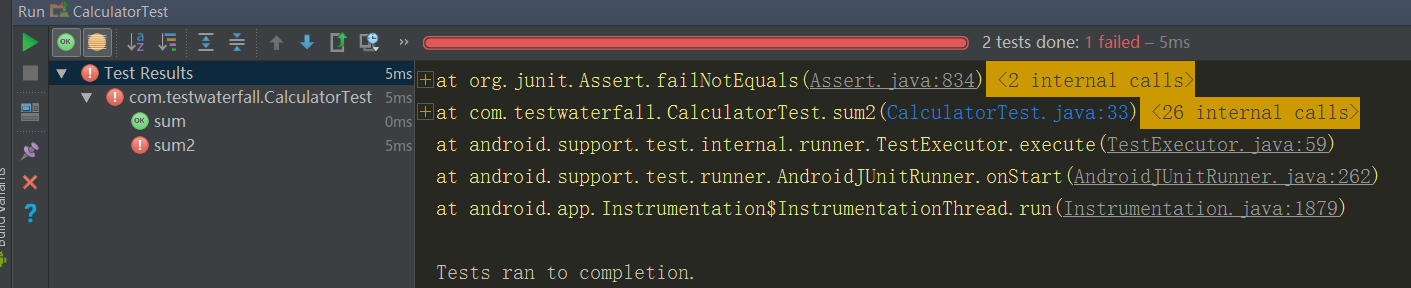# Android Studio中使用junit做单元测试

### 1. 确保你的工程添加了junit依赖，目前一般都会自动添加该项。

dependencies {

testCompile 'junit:junit:4.12'

}

### 3. 假如，我们想要做单元测试的类叫做Calculator，代码如下

public class Calculator {

public int sum(int x,int y){
return x+y;
}

}

### 4. 利用Android Studio图形界面生成测试类的步骤如下所示：

• 在编辑视图中选中当前类Calculator，点击右键，弹出如下界面，点击test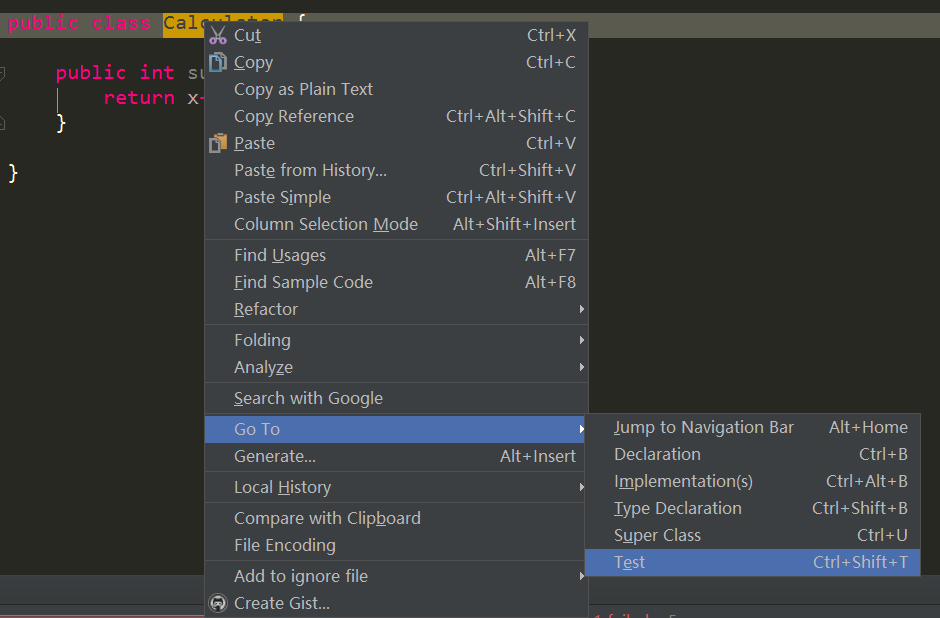• 在接下来的界面中选择Create New Test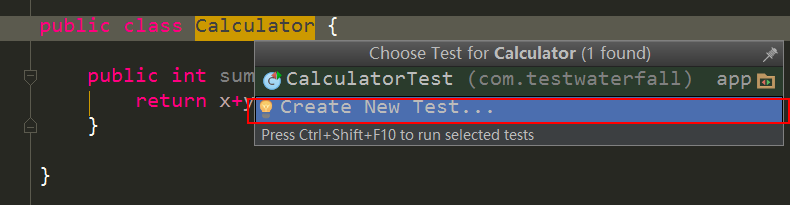• 创建测试类的名字，需要测试的方法和所在的目录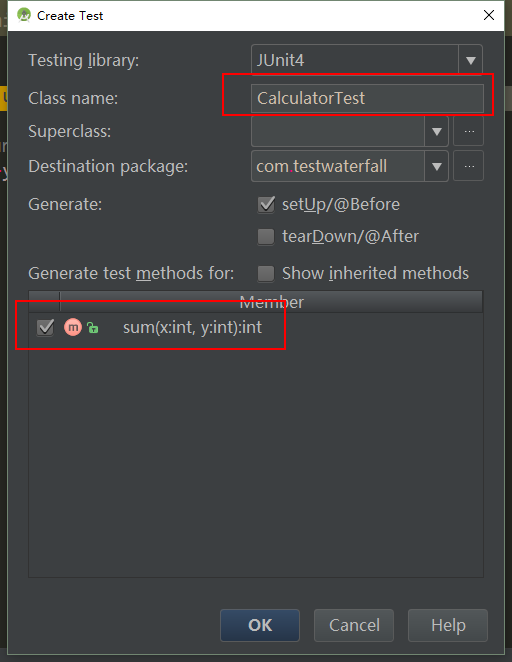• 随便选一个目录作为测试类的目录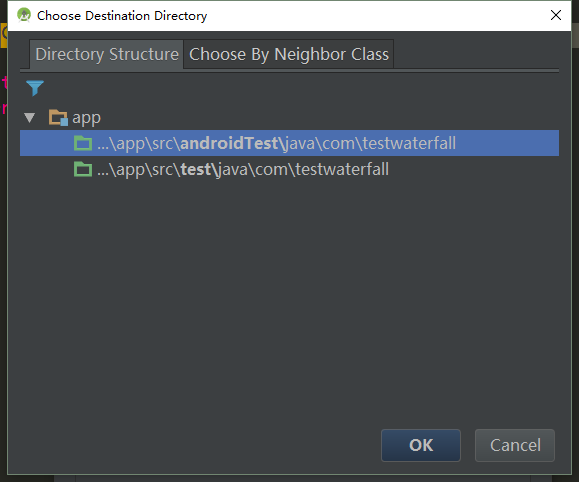• 好，最终生成了一个测试类，它刚开始是这样的
public class CalculatorTest {

@Before
public void setUp() throws Exception {
mCalculator = new Calculator();
}

@After
public void tearDown() throws Exception {

}

@Test
public void sum() throws Exception {

}
}

### 5. 在生成的测试类中编写测试逻辑

• 在测试类中引用需要被测试的对象Calculator，在setUp中给它赋值。
• 在sum方法中编写具体的测试逻辑，如代码所示，检测3+4是不是等于7
• 新添加一个测试方法sum()2，测试3+4是不是等于6（这个显然不能通过测试）
public class CalculatorTest {

private Calculator mCalculator;

@Before
public void setUp() throws Exception {
mCalculator = new Calculator();
}

@After
public void tearDown() throws Exception {

}

@Test
public void sum() throws Exception {
assertEquals(mCalculator.sum(3,4),7);
}

@Test
public void sum2() throws Exception {
assertEquals(mCalculator.sum(3,4),6);
}

}

### 6. 运行单元测试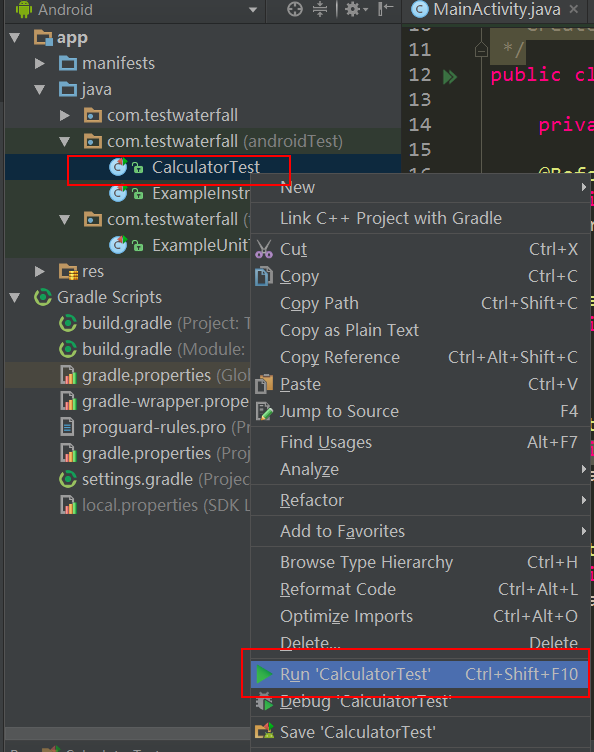### 7. 结果示意图